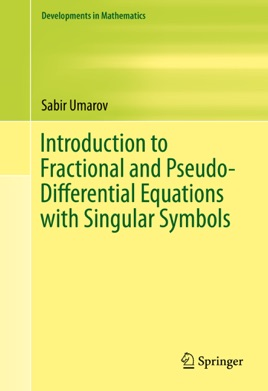• 82,99 €

## Publisher Description

​The book systematically presents the theories of pseudo-differential operators with symbols singular in dual variables, fractional order derivatives, distributed and variable order fractional derivatives, random walk approximants, and applications of these theories to various initial and multi-point boundary value problems for pseudo-differential equations. Fractional Fokker-Planck-Kolmogorov equations associated with a large class of stochastic processes are presented. A complex version of the theory of pseudo-differential operators with meromorphic symbols based on the recently introduced complex Fourier transform is developed and applied for initial and boundary value problems for systems of complex differential and pseudo-differential equations.